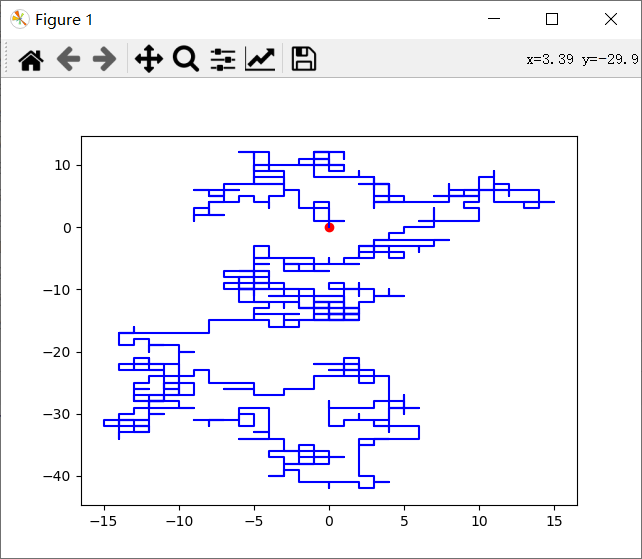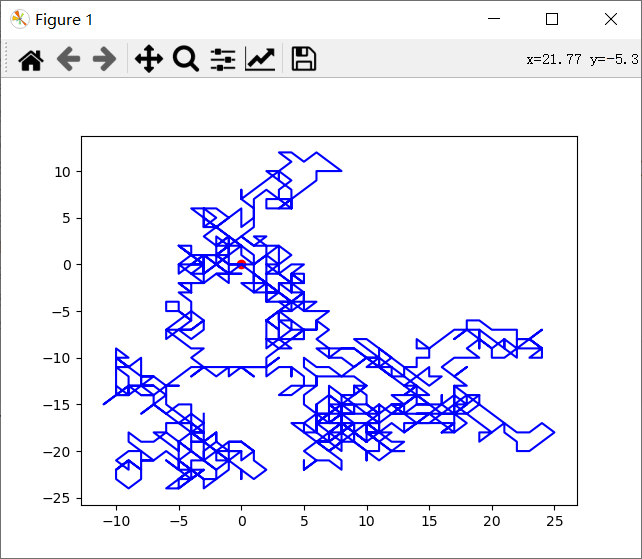﻿ python基础——随机漫步_python_开心洋葱网
• 欢迎访问开心洋葱网站，在线教程，推荐使用最新版火狐浏览器和Chrome浏览器访问本网站，欢迎加入开心洋葱` QQ群`
• 为方便开心洋葱网用户，开心洋葱官网已经开启复制功能！
• 欢迎访问开心洋葱网站，手机也能访问哦~欢迎加入开心洋葱多维思维学习平台` QQ群`
• 如果您觉得本站非常有看点，那么赶紧使用Ctrl+D 收藏开心洋葱吧~~~~~~~~~~~~~！
• 由于近期流量激增，小站的ECS没能经的起亲们的访问，本站依然没有盈利，如果各位看如果觉着文字不错，还请看官给小站打个赏~~~~~~~~~~~~~！

# python基础——随机漫步

2350次浏览 手机上查看

模仿醉汉在二维空间上的随机漫步：一个醉汉喝醉酒，每次只能走一步，每步分别沿着x，y轴走一个单位长度，试着画出醉汉的轨迹。

1. Python 3.8
2. PyCharm Community Edition 2020.3.3 x64

``````import numpy as np
import matplotlib.pyplot as plt
# 定义初始位置坐标 a、b
a = 0
b = 0
# 定义列表 x、y, 为走过的位置记录
x = [a]
y = [b]
# 下一步可以选择的方向
# 四个方向
option = np.array([[0, 1], [1, 0], [0, -1], [-1, 0]])
# 八个方向
# option = np.array([[0, 1], [1, 0], [0, -1], [-1, 0], [1, 1], [1, -1], [-1, -1], [-1, 1]])
# 设置步数
steps = 1000
# 随机漫步
for i in range(steps):
# 随机选择下一步的方向
next = np.random.randint(len(option))
# 更新当前位置
a += np.array(option[next])
b += np.array(option[next])
# 保存到位置记录列表
x.append(a)
y.append(b)
# 画图
# 设置初始点为红色
plt.scatter(0, 0, c='r')
plt.plot(x, y, c='b')
plt.show()
````````````import numpy as np
import matplotlib.pyplot as plt
import math

# 定义初始位置坐标 a、b
a = np.random.randint(10)
b = np.random.randint(10)
# 定义列表 x、y, 为走过的位置记录
x = [a]
y = [b]
# 设置步数
steps = 1000
# 随机漫步
for i in range(steps):
# 随机选择下一步的方向 0 ~ 360 任意方向
next_steps = np.random.randint(360)
# 角度转化为对应的弧度制,便于计算一单位长度 x、y 坐标的变化
angle = np.deg2rad(next_steps)
# 更新当前位置
a += math.cos(angle)
b += math.sin(angle)
# 保存到位置记录列表
x.append(a)
y.append(b)
# 实时画图
# plt.clf() # 清除之前画的图
# plt.scatter(x, y, c='r')
# plt.plot(x, y, c='b')
# plt.pause(0.1) # 暂停多少秒
# plt.ioff()
# 画图，设置初始点为红色
plt.scatter(x, y, c='r')
plt.plot(x, y, c='b')
plt.show()
``````从结果可以看出不管是四个方向移动，还是八个方向移动，还是任意方向移动的，整体的效果都是无序的，因为在每一步选择方向的时候，都是随机选择的，使得下一步是不确定的，所以整体上看都是无序的。

[开心洋葱]• 版权声明

本站的文章和资源来自互联网或者站长的原创，按照 CC BY -NC -SA 3.0 CN协议发布和共享，转载或引用本站文章应遵循相同协议。如果有侵犯版权的资源请尽快联系站长，我们会在24h内删除有争议的资源。
• 合作网站

• 友情链接

• 关于我们

一群热爱思考，热爱生活，有理想的新社会主义接班人的多维思维学习平台，天行健，君子以自强不息。地势坤，君子以厚德载物。
……